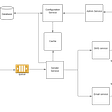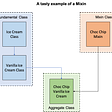# My First Haskell Program (Part 1)

• It is a “purely functional language”. This means that any function written in Haskell if called with the same arguments will return the same values, and does not have any “side effects”. This means that it will not modify an array or map, print anything out or do any other kind of I/O.
• It has a very powerful system of static types.

# The familiar and easy part

`# "10" here is the max line length to use, and words.txt is# a file containing the words to print.\$ ./consistent_printer 10 words.txt`
`main = do  [lineLengthRaw, fileName] <- getArgs`
`main = do   [lineLengthRaw, fileName] <- getArgs  putStr lineLengthRaw  putStr fileName`

# Inverting Control Flow

`for i in xrange(len(words)):    minimum_cost = sys.maxsize    for j in xrange(i + 1):        line_length = len(" ".join(words[j:i+1]))        if not line_length > max_line_length:            extra_space = max_line_length - line_length            previous_cost = 0 if j == 0 else cost_array[j - 1]            total_cost = (previous_cost + (extra_space ** 2))            if total_cost < minimum_cost:                minimum_cost = total_cost     cost_array[i] = minimum_cost`
`-- `costOf` refers to the minimum cost if linebreak-- is placed after the ith word.costOf :: [String] -> Int -> Int -> IntcostOf list max_line_length i  | i == 0 = 0  | otherwise = minimum costAtEachJ  where costAtEachJ = map (costAtJ list max_line_length i) [0..i-1]`
`-- `costAtJ` is the cost incurred by placing a newline-- after j.costAtJ :: [String] -> Int -> Int -> Int -> IntcostAtJ list max_line_length i j  | lineLength > max_line_length = (maxBound :: Int)  | otherwise = previous_cost + extra_space ^ 2  where lineLength = length (intercalate " " (drop j (take i list)))        previous_cost :: Int        previous_cost          | j == 0 = 0          | otherwise = costOf j list max_line_length        extra_space = max_line_length - lineLength`

# Conclusion

--

--

--

## More from Sidharth Shanker

Software Engineer @fin

Love podcasts or audiobooks? Learn on the go with our new app.

## Designing a scalable Notification Service## Are Your Discovery Tools Showing You the Big Picture of Your IT Ecosystem?## Cloud Firestore## Why I like Rust?## [Frequently asked Product Management Interview Question] What is your favorite software Product## Peter’s Adventures in Ruby: Garbage Collection in Ruby## Sidharth Shanker

Software Engineer @fin

## Fundamentals of functional programming## What is a Bug?## Mixins in Common Lisp## Haskell Journey: Higher Order Functions